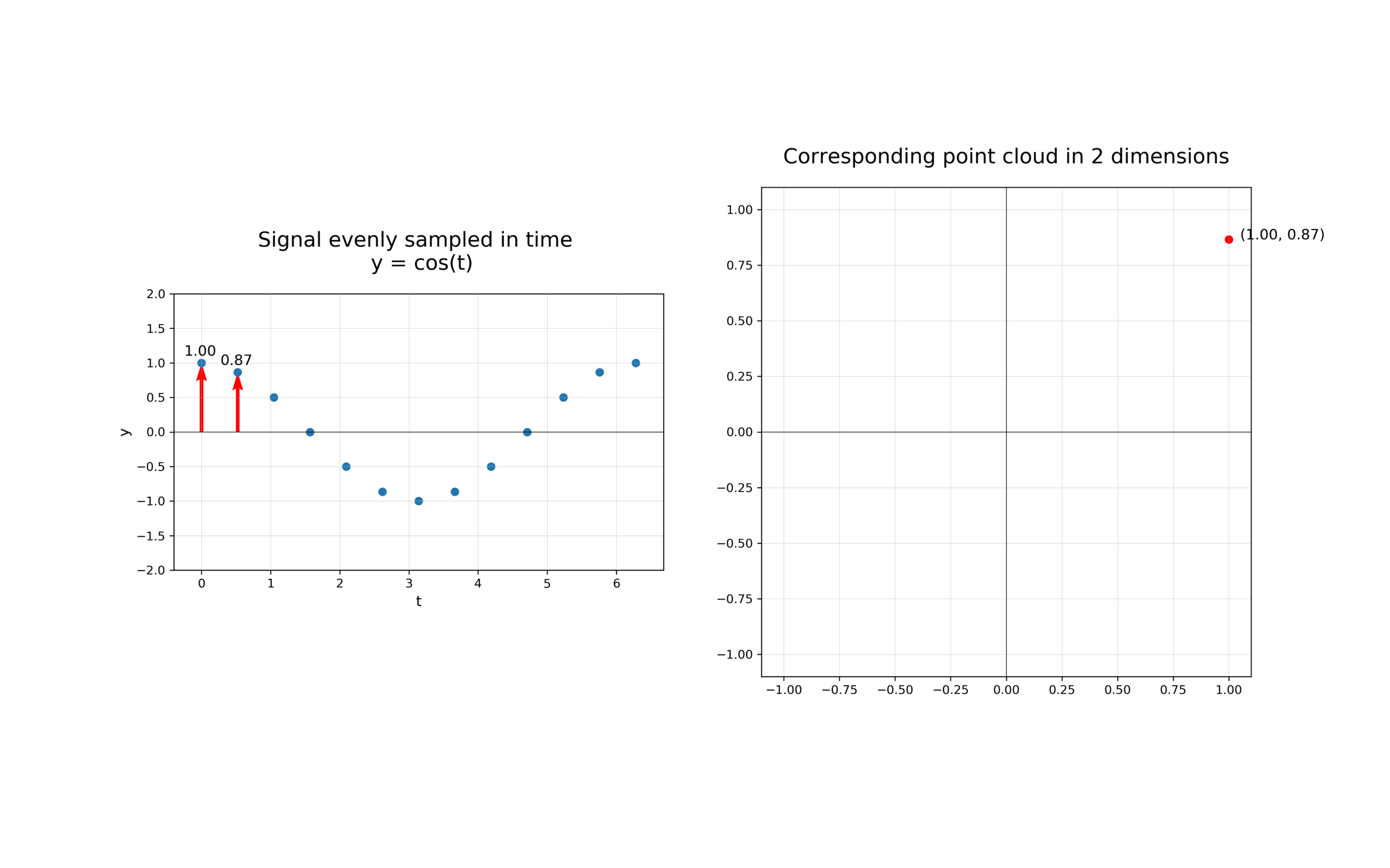Topology of time series¶

This notebook explores how giotto-tda can be used to gain insights from time-varying data by using ideas from from dynamical systems and persistent homology.

If you are looking at a static version of this notebook and would like to run its contents, head over to GitHub and download the source.

From time series to time delay embeddings¶

The first step in analysing the topology of time series is to construct a time delay embedding or Takens embedding, named after Floris Takens who pioneered its use in the study of dynamical systems. A time delay embedding can be thought of as sliding a “window” of fixed size over a signal, with each window represented as a point in a (possibly) higher-dimensional space. A simple example is shown in the animation below, where pairs of points in a 1-dimensional signal are mapped to coordinates in a 2-dimensional embedding space.A 2-dimensional time delay embedding

More formally, given a time series $$f(t)$$, one can extract a sequence of vectors of the form $$f_i = [f(t_i), f(t_i + \tau), f(t_i + 2 \tau), \ldots, f(t_i + (d-1) \tau)] \in \mathbb{R}^{d}$$, where $$d$$ is the embedding dimension and $$\tau$$ is the time delay. The quantity $$(d-1)\tau$$ is known as the “window size” and the difference between $$t_{i+1}$$ and $$t_i$$ is called the stride. In other words, the time delay embedding of $$f$$ with parameters $$(d,\tau)$$ is the function

$\begin{split}TD_{d,\tau} f : \mathbb{R} \to \mathbb{R}^{d}\,, \qquad t \to \begin{bmatrix} f(t) \\ f(t + \tau) \\ f(t + 2\tau) \\ \vdots \\ f(t + (d-1)\tau) \end{bmatrix}\end{split}$

and the main idea we will explore in this notebook is that if $$f$$ has a non-trivial recurrent structure, then the image of $$TD_{d,\tau}f$$ will have non-trivial topology for appropriate choices of $$(d, \tau)$$.

A periodic example¶

As a warm-up, recall that a function is periodic with period $$T > 0$$ if $$f(t + T) = f(t)$$ for all $$t \in \mathbb{R}$$. For example, consider the function $$f(t) = \cos(5 t)$$ which can be visualised as follows:

import numpy as np
import plotly.graph_objects as go

x_periodic = np.linspace(0, 10, 1000)
y_periodic = np.cos(5 * x_periodic)

fig = go.Figure(data=go.Scatter(x=x_periodic, y=y_periodic))
fig.update_layout(xaxis_title="Timestamp", yaxis_title="Amplitude")
fig.show()